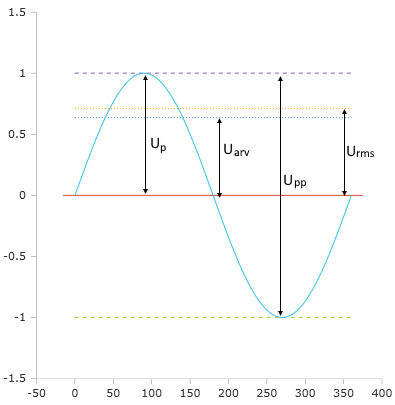# Calculate AC voltage parameters

Online calculator and formulas for calculating effective and mean values for alternating voltage

## Calculate AC voltage online

From the specified voltage, this function calculates the sinusoidal parameters for the rms voltage, peak voltage, peak-to-peak voltage and rectification voltage. The rms voltage is preset for the input. The type of input voltage can be changed in the menu.

AC voltage calculator

 Input RMS voltage Peak voltage Peak / peak voltage Rectifying voltage Decimal places 0 1 2 3 4 6 8 10 Result RMS voltage Peak voltage Peak-to-peak voltage Rectifying voltage

## Parameters and formulasWhen voltage is generated in a rotating generator, a sinusoidal and at the same time periodically repeating alternating voltage is generated.

### Nominal value and effective value

The effective value of an alternating voltage $$\displaystyle U_{rms}$$ is the value that generates the same heat in a resistor as a DC voltage of the same size. The term "230 V" for the normal household AC voltage is the effective value.

If the peak value is known, the rms value can be calculated using the following formula:

$$\displaystyle U_{rms}=\frac{U_p}{\sqrt{2}}$$

### Maximum value, peak value

The peak value for a sinusoidal voltage is the highest voltage level that can be achieved. With a given effective value, the peak value can be calculated using the following formula:

$$\displaystyle U_p=U_{rms}·\sqrt{2}$$

### Peak-to-peak voltage

The peak-to-peak voltage $$\displaystyle U_{pp}$$ is the difference between the positive and negative peak value and thus twice the peak value.

$$\displaystyle U_{pp}=2 ·U_p$$

### Rectified value

The rectified value is the arithmetic mean value of the rectified AC voltage. In the case of pure sinusoidal voltages, it can easily be calculated by dividing the rms voltage by 1,111.

$$\displaystyle U_{arv}= \frac{U_{rms}}{1.11}$$

### Form factor

The form factor indicates the ratio of the effective value to the rectified value.
With sinusoidal alternating voltage, it is 1.111 (exactly $$\displaystyle \frac{π} {\sqrt{8}}$$).

### Crest factor

The crest factor is the ratio of the peak value to the rms value.
With a sinusoidal voltage, the crest factor is 1.414 (exactly $$\displaystyle \sqrt{2}$$ ).

#### Legend

 $$\displaystyle U_{rms}$$ Effective voltage $$\displaystyle U_{p}$$ Peak voltage $$\displaystyle U_{pp}$$ Peak to peak voltage $$\displaystyle U_{arv}$$ Rectified value